# Arithmetic Operators in Oracle

## Arithmetic Operators in Oracle with Examples

In this article, I am going to discuss Arithmetic Operators in Oracle with Examples. Please read our previous article, where we discussed Data Query Language (DQL) or Data Retrieve Language (DRL) command in Oracle with Examples. Before understanding Arithmetic Operators, let us first try to understand what are operators and their types.

##### What are Operators in Oracle?

Operators are used to expressing the conditions in Select statements. The operator manipulates individual data items and returns a result. The data items are called operands or arguments. The different types of Operators available in Oracle are as follows:

• Arithmetic operators
• Assignment operator
• Relational operators
• Logical operators
• Special Operators
• Set Operators

Note: In this article, we are going to discuss Arithmetic Operators in Oracle with Examples, and the rest operators are going to be discussed in our next subsequent articles.

##### Example to Understand Arithmetic Operators in Oracle:

We are going to use the following Employee table to understand the need and use of Arithmetic Operator in Oracle with Examples.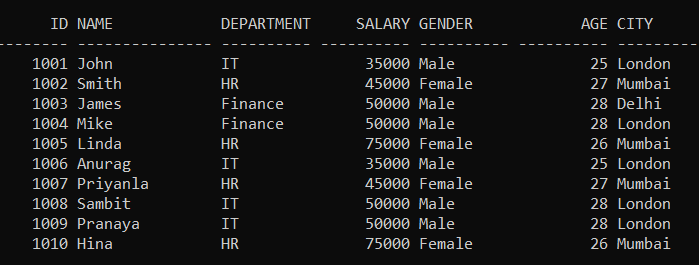Please execute the below SQL query to drop the existing Employee table and Create a new Employee table with the required sample data. We also set the linesize to get the output in the above format.

```SET linesize 300;

DROP Table Employee;

CREATE TABLE Employee (
Id INT PRIMARY KEY,
Name VARCHAR(15),
Department VARCHAR(10),
Salary NUMBER(8, 2),
Gender VARCHAR(10),
Age INT,
City VARCHAR(10)
);

INSERT INTO Employee (Id, Name, Department, Salary, Gender, Age, City) VALUES (1001, 'John', 'IT', 35000, 'Male', 25, 'London');
INSERT INTO Employee (Id, Name, Department, Salary, Gender, Age, City) VALUES (1002, 'Smith', 'HR', 45000, 'Female', 27, 'Mumbai');
INSERT INTO Employee (Id, Name, Department, Salary, Gender, Age, City) VALUES (1003, 'James', 'Finance', 50000, 'Male', 28, 'Delhi');
INSERT INTO Employee (Id, Name, Department, Salary, Gender, Age, City) VALUES (1004, 'Mike', 'Finance', 50000, 'Male', 28, 'London');
INSERT INTO Employee (Id, Name, Department, Salary, Gender, Age, City) VALUES (1005, 'Linda', 'HR', 75000, 'Female', 26, 'Mumbai');
INSERT INTO Employee (Id, Name, Department, Salary, Gender, Age, City) VALUES (1006, 'Anurag', 'IT', 35000, 'Male', 25, 'London');
INSERT INTO Employee (Id, Name, Department, Salary, Gender, Age, City) VALUES (1007, 'Priyanla', 'HR', 45000, 'Female', 27, 'Mumbai');
INSERT INTO Employee (Id, Name, Department, Salary, Gender, Age, City) VALUES (1008, 'Sambit', 'IT', 50000, 'Male', 28, 'London');
INSERT INTO Employee (Id, Name, Department, Salary, Gender, Age, City) VALUES (1009, 'Pranaya', 'IT', 50000, 'Male', 28, 'London');
INSERT INTO Employee (Id, Name, Department, Salary, Gender, Age, City) VALUES (1010, 'Hina', 'HR', 75000, 'Female', 26, 'Mumbai');
```
##### Arithmetic Operators in Oracle:

The Arithmetic Operators in Oracle are used for performing mathematical calculations such as Addition, Subtraction, Multiplication, Module, and Division represented by the expected +, -, *(star or asterisk), %, and / forward slash symbols respectively on the given operand values. That is, they are used to perform mathematical operations on two expressions of the same or different data types of numeric data.

1. The arithmetic operations can be used to create expressions on number and date data.
2. The arithmetic operations can be Used to perform any Arithmetic Operations like Addition, Subtraction, Multiplication, and Divided by.
3. The arithmetic operators can be used in any clause of a SQL statement.
4. SQL * Plus ignores the blank spaces before and after the arithmetic operator.

The arithmetic operators can be directly used in the SQL statement or in combination with column values. The different arithmetic operators supported by MySQL are shown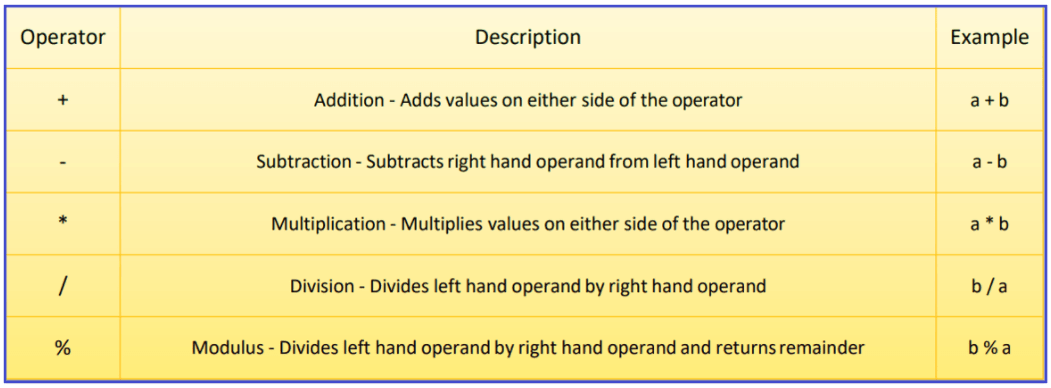##### How Arithmetic Operators are Evaluated in Oracle?

Similar to basic arithmetic calculations, the arithmetic operators in Oracle also have the same Operator Precedence. If the arithmetic expression contains more than one operator, then the multiplication and division operators are the highest priority and are evaluated first, and then the addition and minus (subtraction) operators are evaluated.

When two operators are having the same priority, then the expression is going to be evaluated from left to right in the Oracle database. The Parentheses in Oracle can also be used to force an operation to take priority over any other operators. Parentheses are also used to improve code readability.

Example: Display salary of employees with 2000 increments in their salary.

SELECT ID, Name, Salary, Salary + 2000 “Incremented salary” FROM Employee;

Explanation: In the Employee table every employee’s salary sums it 2000. When you execute the above query, you will get the following output.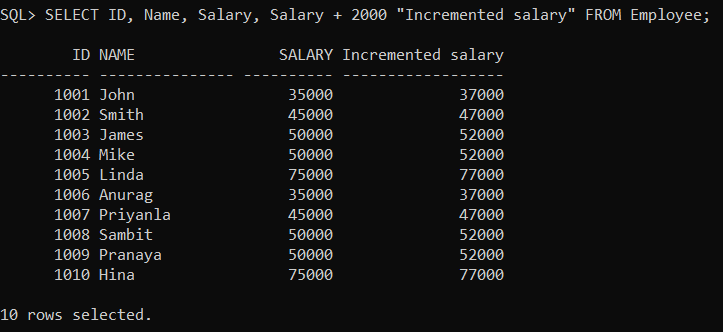##### Arithmetic Operator Subtraction (-):

This operator is used to perform the subtraction between two numbers and dates.

Example: Display the details of employees decreasing their salary by 200.

SELECT ID, Name, Salary, Salary – 200 “Decreased Salary” FROM Employee;

Explanation: In the Employee table every employee’s salary is subtracted with 200. When you execute the above SQL query, you will get the following output.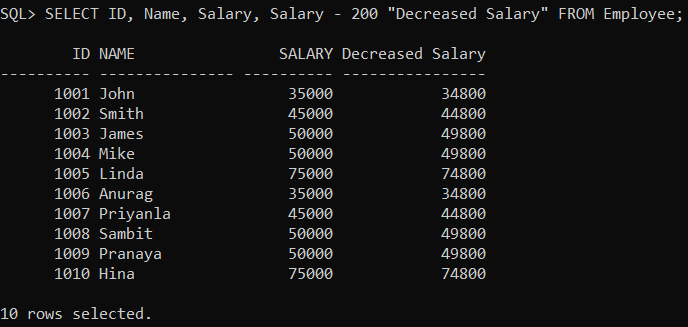##### Arithmetic Operator Multiplication (*):

This operator is used to perform multiplication.

Example: Display the details of the employees Incrementing their salary two times.

SELECT ID, Name, Salary, Salary * 2 “Increased Salary” FROM Employee;

Explanation: Every Employee’s table salary is multiplied by 2. When you execute the above SQL query, you will get the following output.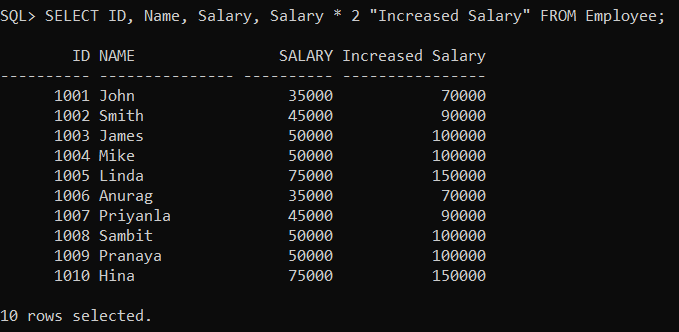##### Arithmetic Operator Division (/):

This operator is used to perform a Division test. The division will display only the Quotient value, not the remainder value. Example 6/2 gives 3 because 2 divides 6 by 3 times.

Example: Display half of the salary of employees.

SELECT ID, Name, Salary, Salary / 2 “Division Salary” FROM Employee;

When you execute the above SQL query, you will get the following output.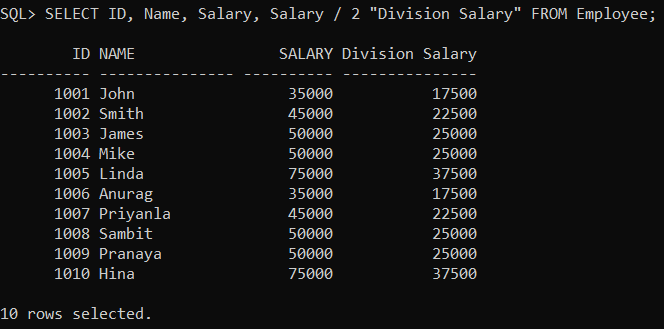###### More Examples:
```SELECT ID, Name, 12*Salary+100 "Salary" FROM Employee;
SELECT ID, Name, (12*Salary)+100 "Salary" FROM Employee;
SELECT ID, Name, 12*(Salary+100) "Salary" FROM Employee;```
##### Arithmetic Operations on String Values in Oracle

In oracle, it is not possible to perform any calculations on String values (such as Varchar or Char). The following SQL statement will give you an error.

SELECT ID, Name + 100 FROM Employee;

When you try to execute the above code, you will get the following error.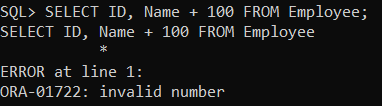In the next article, I am going to discuss Assignment Operators in Oracle with Examples. Here, in this article, I try to explain Arithmetic Operators in Oracle with Examples and I hope you enjoy this Arithmetic Operators in Oracle with Examples article.